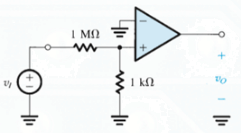# Test: The Ideal Operational Amplifiers

## 10 Questions MCQ Test Analog Circuits | Test: The Ideal Operational Amplifiers

Description
Attempt Test: The Ideal Operational Amplifiers | 10 questions in 10 minutes | Mock test for Electronics and Communication Engineering (ECE) preparation | Free important questions MCQ to study Analog Circuits for Electronics and Communication Engineering (ECE) Exam | Download free PDF with solutions
QUESTION: 1

### What is the minimum number of terminals required in an IC package containing four operational amplifiers (quad op amps)?

Solution:

The minimum no of pins required by dual-op-amp is 8. Each op-amp has 2 input terminals(4 pins) and one output terminal(2 pins). Another 2 pins are required for power.
Similarly, The minimum no of pins required by dual-op-amp is 14: 4*2 + 4*1 + 2 = 14.

QUESTION: 2

### Which of the following is not a property of an ideal operational amplifier?

Solution:

An ideal operational amplifier does not has a zero input impedance.

QUESTION: 3

### In an ideal op amp the open-loop gain is 103. The op amp is used in a feedback circuit, and the voltages appearing at two of its three signal terminals are measured as v2 = 0V and v3 = 2V where it is assumed that v1 and v2 are input terminals and v3 is the output terminal. The value of the differential (vd) and common-mode (vcm)signal is

Solution:

Vc = 0.5(V1 + V2) and
Vd = V2 – V1.

QUESTION: 4

Consider the figure given below. Known that vo = 4V and vi = 2V, determine the gain for the op amp assuming that it is ideal except for the fact that it has finite gain​Solution:

The Voltage at the positive input has to be -3.000v, vi = -3.020v
A = vo / vi – vr = -2 / -3.020 -(-3) = 100.

QUESTION: 5

Which of the following is not a terminal for the operational amplifier?

Solution:

There are three terminals for the operational amplifier.

QUESTION: 6

Operational amplifiers are

Solution:

It is another way to refer to op amps based on its terminal characteristics.

QUESTION: 7

Express the input voltages v1 and v2 in terms of differential input (vd) and common-mode input(vc). Given v2 > v2.

Solution:

This is the correct mathematical representation.

QUESTION: 8

What is the minimum number of pins for a dual operational amplifier IC package?

Solution:

The minimum no of pins required by dual-op-amp is 8. Each op-amp has 2 input terminals(4 pins) and one output terminal(2 pins). Another 2 pins are required for power.

QUESTION: 9

For an ideal operational amplifier (except for the fact that it has finite gain) one set of the value for the input voltages (v2 is the positive terminal v1 is the negative terminal) and output voltage (v0) as determined experimentally is v1= 2.01V, v2=2.00V and v0= -0.99V. Experiment was carried with different values of input and output voltages. Which of the following is not possible considering experimental error?

Solution:

Only option d does not satisfies the mathematical relation between the given quantities.

QUESTION: 10

What are the units of slew rate?

Solution:

These units are obtained from the definition of the term slew rate.Use Code STAYHOME200 and get INR 200 additional OFF Use Coupon Code